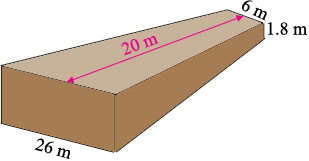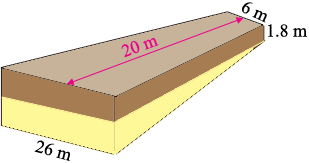SEARCH HOMEMath Central Quandaries & QueriesQuestion from steve, a parent: hi i have a large ditch to make a road entrance across, the ditch is a green field on one side and a highway on the other side the ditch depth is 1.8m on the field side and 3.2 on the highway side the culvert pipe is 1,2m in diameter are you with me so far? the entrance from the highway will be 26m wide tapering in to 6m - ,20 m in from the highway there will be a concrete slab of 150mm over the top the fall from highway to field must not exceed 1/8 any ideas how much stone it will needHi Steve,

This is a big hole you need to fill!

Here is my diagram, it is very much not to scale.I am going to partition the volume into two parts as in the diagram below.The top piece, shaded brown is a trapezoidal prism and its volume is

$\frac12 (26 + 6) \times 20 \times 1.8 = 576 \mbox{ cubic metres.}$

The piece shaded yellow is a wedge and its volume is

$\frac16 \times 1.4 \times 20 \times (52 + 6) = 271 \mbox{ cubic metres.}$

assume that the culvert pipe is parallel to the highway and is about halfway from the highway to the field. This it need to be about 16 m long and hence its volume is

$\pi \frac{1.2}{2} \times 16 = 18 \mbox{ cubic metres.}$

Thus the volume of stone you need is approximately

$526 + 271 - 18 = 829 \mbox{ cubic metres.}$

HarleyMath Central is supported by the University of Regina and The Pacific Institute for the Mathematical Sciences.